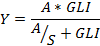SORTIE-ND
Software for spatially-explicit simulation of forest dynamics

# Relative growth behaviors

Several behaviors apply a relative growth version of the Michaelis-Menton function.

### Parameters for these behaviors

Parameter nameDescription
Adult Constant Area Growth in sq. cm/yrThe constant amount of basal area by which to increase a tree's basal area. Applies to basal-area-increment-limited behaviors.
Asymptotic Diameter Growth (A)Asymptote of the Michaelis-Menton growth function at high light - function term A below; for diameter growth.
Asymptotic Height Growth (A)Asymptote of the Michaelis-Menton growth function at high light - function term A; for height growth.
Slope of Growth Response (S)Slope of the Michaelis-Menton growth function at zero light - function term S below.
Relative Michaelis-Menton Growth - Diameter ExponentThe exponent to be used with diameter when calculating relative growth.

Relative growth is calculated with the equation:where:
• Y is the amount of annual relative growth
• A is the Asymptotic Diameter Growth (A) or Asymptotic Height Growth (A) parameter
• S is the Slope of Growth Response (S) or Slope of Height Growth Response (S) parameter
• GLI is the global light index, calculated by a light behavior

Diameter growth is compounded over multiple timesteps with the equation:

G = ((Y + 1)T - 1) * diam X
where:
• G is the amount of diameter growth for the timestep, in cm
• diam is the diameter of the tree in cm (at 10 cm height if seedling or sapling, or DBH if adult)
• T is the number of years per timestep
• X is the Relative Michaelis-Menton Growth - Diameter Exponent parameter

Relative height growth is calculated slightly differently. The details are discussed in the section for the Relative growth - height only behavior below. Relative growth is discussed in Pacala et al 1996.

## Relative growth limited to radial increment

### How it works

This behavior calculates an amount of diameter growth according to the relative growth equation. Growth is limited to a maximum of the constant radial growth increment for the species of tree to which it is being applied. The increment is calculated as described in the "Constant radial growth" behavior. Note that the increment parameter specifies radial growth; the behavior makes all necessary conversions.

### How to apply it

This behavior can be applied to seedlings, saplings, and adults of any species. Any tree species/type combination to which it is applied must also have a light behavior applied. You can use either the diam with auto height or diam only version.

## Relative growth limited to basal area increment

### How it works

This behavior calculates an amount of diameter growth according to the relative growth equation. Growth is limited to a maximum of a constant basal area increment. The amount of diameter increase is calculated by dividing the annual basal area increment of the tree's species by the diameter of the tree. The increment is calculated as described in the "Constant basal area growth" behavior.

### How to apply it

This behavior can be applied to seedlings, saplings, and adults of any species. Any tree species/type combination to which it is applied must also have a light behavior applied. You can use either the diam with auto height or diam only version.

## Non-limited relative growth

### How it works

The amount of increase returned by the relative growth equation is applied to the tree.

### How to apply it

This behavior can be applied to seedlings, saplings, and adults of any species. Any tree species/type combination to which it is applied must also have a light behavior applied.

## Relative growth - height only

This behavior uses the Michaelis-Menton function to do height growth.

### How it works

After the Michaelis-Menton function is used to calculate Y as described in the section above, the amount of height growth is calculated as:

G = Y * Height X

where:

• G is the amount of height growth for one year, in cm
• Height is the height of the tree in cm
• X is the Relative Michaelis-Menton Growth - Height Exponent parameter

If the timestep is more than one year long, growth is recalculated for each year of the timestep, increasing the height each time.

### How to apply it

This behavior can be applied to seedlings, saplings, and adults of any species. Any tree species/type combination to which it is applied must also have a light behavior and a diameter growth behavior applied.